# python机器学习手写算法系列——线性回归

http://blog.csdn.net/juwikuang/article/details/73057194

# 从机器学习到线性回归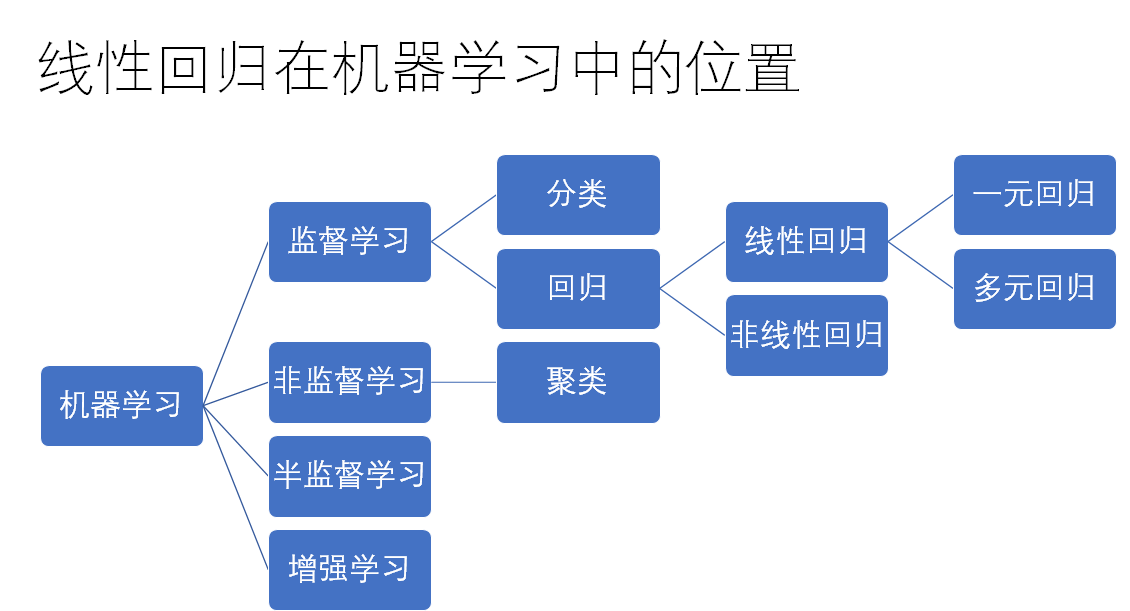# 问题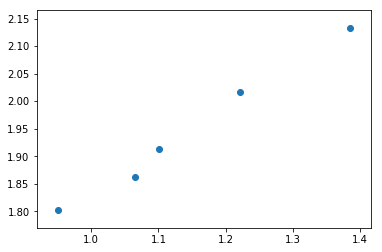# 数学模型

## 一元线性回归公式

$y=ax+b+ε$

y 为应变量 dependent variable
x 为自变量 independent variable
a 为斜率 coeffient
b 为截距 intercept
ε （读作epsilon）为误差，正态分布

$\hat{y}=ax+b$
$ε=y-\hat{y}$

$\hat{y}$ 读作y hat，也有人读作y帽子。这里的帽子一般表示估计值，用来区别真实值y。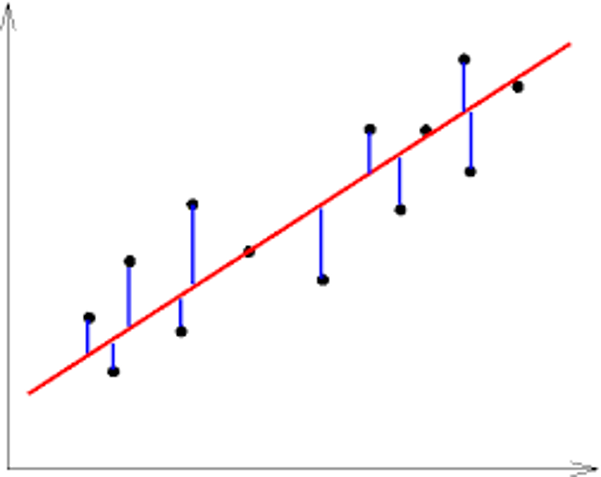（图片来自互联网）

x红色的线为回归线，即$\hat{y}=ax+b$

x蓝色的线段为误差，即$ε=y-\hat{y}$

## 方差 - 损失函数 Cost Function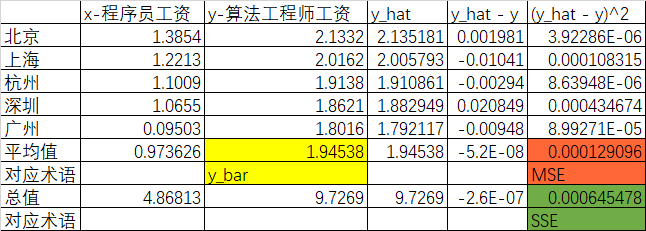$J(a,b)=\frac{1}{2n}\sum_{i=0}^{n}(y_i−\hat{y}_i )^2$

## 优化方法 Optimization Function

$\frac{\partial J}{\partial a} = \frac{\partial \frac{1}{2n}\sum_{i=0}^{n}(y_i−\hat{y}_i )^2}{\partial a}$
$= \frac{1}{n}\sum_{i=0}^{n}(y_i-ax_i-b) \frac{\partial (y_i-ax_i-b)}{\partial a}$
$= \frac{1}{n}\sum_{i=0}^{n}(y_i-ax_i-b) (-x_i)$
$= \frac{1}{n}\sum_{i=0}^{n}x(\hat{y}_i-y_i)$

$\frac{\partial J}{\partial b} = \frac{\partial \frac{1}{2n}\sum_{i=0}^{n}(y_i−\hat{y}_i )^2}{\partial a}$
$= \frac{1}{n}\sum_{i=0}^{n}(y_i-ax_i-b) \frac{\partial (y_i-ax_i-b)}{\partial b}$
$= \frac{1}{n}\sum_{i=0}^{n}(y_i-ax_i-b) (-1)$
$= \frac{1}{n}\sum_{i=0}^{n}(\hat{y}_i-y_i)$

$a = a - \alpha \frac{\partial J}{\partial a}$
$b = b - \alpha \frac{\partial J}{\partial b}$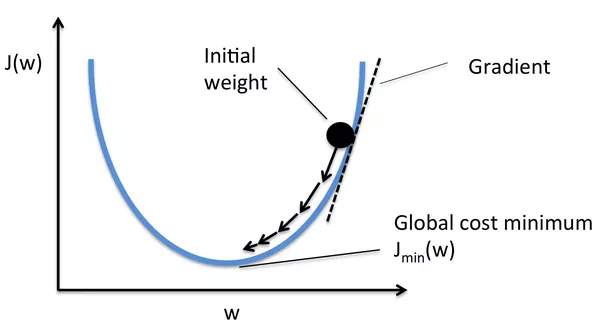（图片来自互联网）

## 算法步骤

1. a和b的起始值设置为零
2. 通过模型$\hat{y}=ax+b$，我们可以算出$\hat{y}$
3. 有了$\hat{y}$，就可以用优化方法算去更新参数
4. 重复2和3，直到找到J的最小值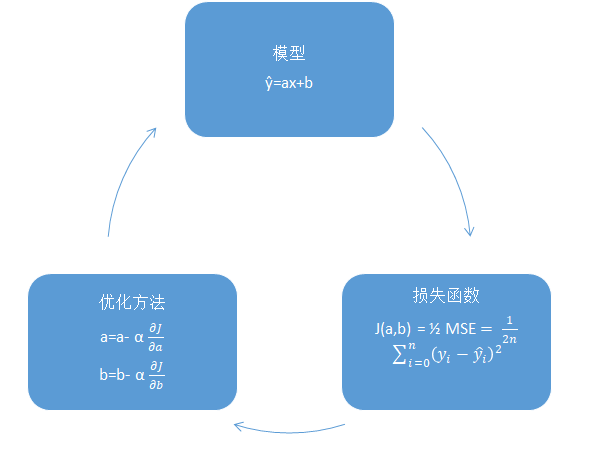# Python 实现

def model(a, b, x):
return a*x + b


def cost_function(a, b, x, y):
n = 5
return 0.5/n * (np.square(y-a*x-b)).sum()


def optimize(a,b,x,y):
n = 5
alpha = 1e-1
y_hat = model(a,b,x)
da = (1.0/n) * ((y_hat-y)*x).sum()
db = (1.0/n) * ((y_hat-y).sum())
a = a - alpha*da
b = b - alpha*db
return a, b


## 训练模型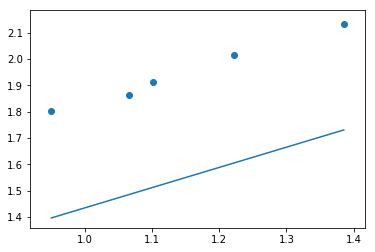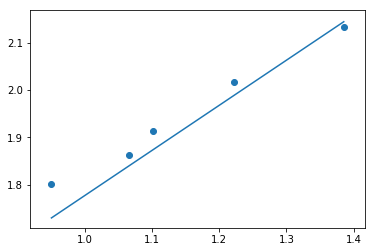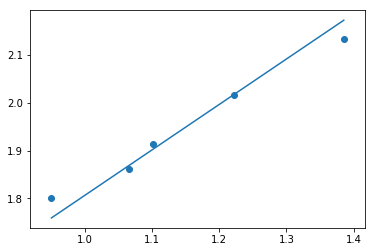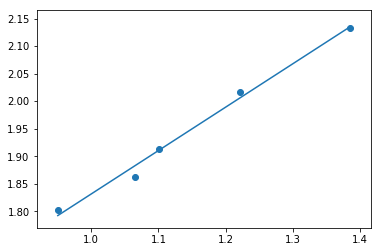## 模型评价

$R^2=SSR/SST$

$SSR=\sum_{i=0}^{n}(\hat{y}_i-\bar{y})$
$SST=\sum_{i=0}^{n}(y_i-\bar{y})$
$\bar{y}$ 读作y bar，是y的平均值。

## 用scikit-learn训练和评价模型

http://scikit-learn.org/

import numpy as np
from sklearn.linear_model import LinearRegression
import matplotlib.pyplot as plt
%matplotlib inline

x = [13854,12213,11009,10655,9503] #程序员工资，顺序为北京，上海，杭州，深圳，广州
x = np.reshape(x,newshape=(5,1)) / 10000.0
y =  [21332, 20162, 19138, 18621, 18016] #算法工程师，顺序和上面一致
y = np.reshape(y,newshape=(5,1)) / 10000.0
# 调用模型
lr = LinearRegression()
# 训练模型
lr.fit(x,y)
# 计算R平方
print lr.score(x,y)
# 计算y_hat
y_hat = lr.predict(x)
# 打印出图
plt.scatter(x,y)
plt.plot(x, y_hat)



# python机器学习手写算法系列

https://github.com/juwikuang/machine_learning_step_by_step

《python机器学习手写算法系列——线性回归》

《python机器学习手写算法系列——逻辑回归》

《python机器学习手写算法系列——决策树》

《python机器学习手写算法系列——kmeans聚类》